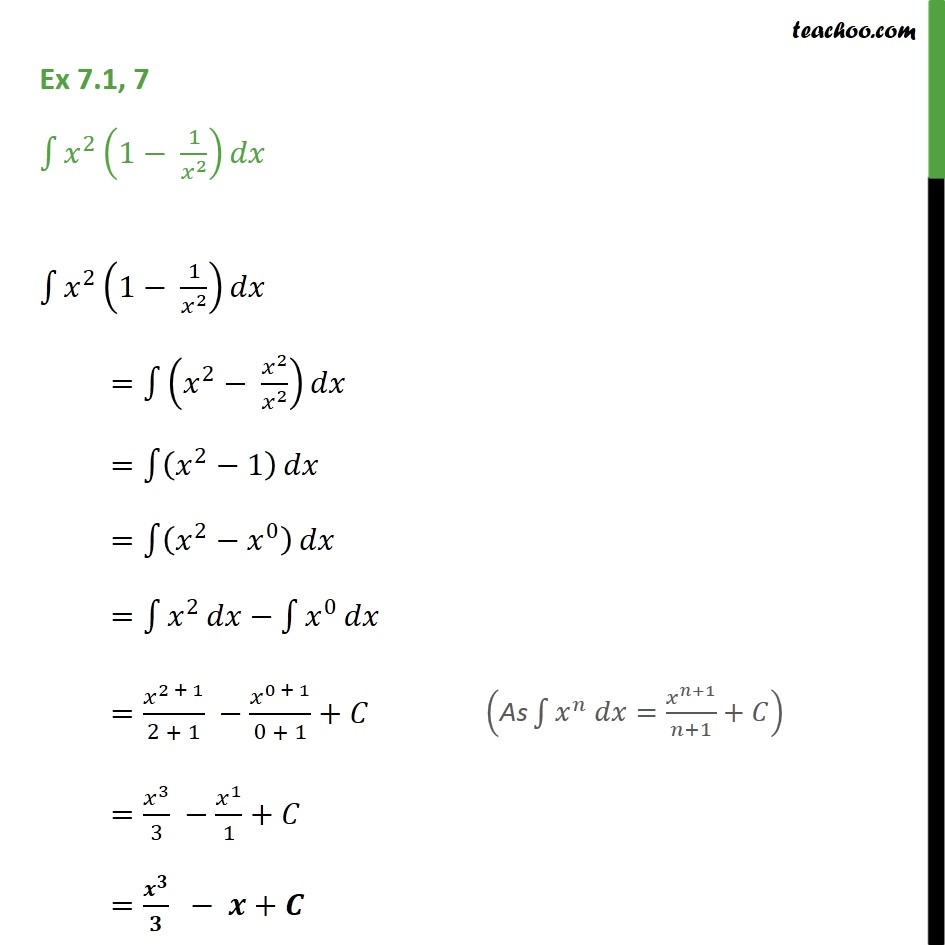Ex 7.1

Chapter 7 Class 12 Integrals
Serial order wiseGet live Maths 1-on-1 Classs - Class 6 to 12

### Transcript

Ex 7.1, 7 - Chapter 7 Class 12 Integrals - NCERT Solution Integrate ∫1▒〖𝑥^2 (1− 1/𝑥^2 ) 〗 𝑑𝑥 ∫1▒〖𝑥^2 (1− 1/𝑥^2 ) 〗 𝑑𝑥 =∫1▒(𝑥^2− 𝑥^2/𝑥^2 ) 𝑑𝑥 =∫1▒(𝑥^2−1) 𝑑𝑥 =∫1▒(𝑥^2−𝑥^0 ) 𝑑𝑥 =∫1▒𝑥^2 𝑑𝑥−∫1▒𝑥^0 𝑑𝑥 =𝑥^(2 + 1)/(2 + 1) −𝑥^(0 + 1)/(0 + 1)+𝐶 ("As" ∫1▒〖𝑥^𝑛 𝑑𝑥=𝑥^(𝑛+1)/(𝑛+1)+𝐶〗) =𝑥^3/3 −𝑥^1/1+𝐶 =𝒙^𝟑/𝟑 − 𝒙+𝑪